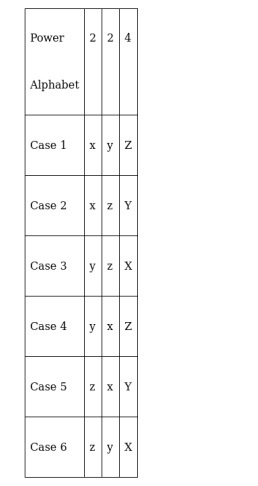# In how many ways can the letters of the expression

Question:

In how many ways can the letters of the expression $x^{2} y^{2} z^{4}$ be arranged when written without using exponents?

Solution:

To find: number of ways the letters can be arranged

The following table shows the possible arrangementsHowever, we see that case $1=x^{2} y^{2} z^{4}$ is the same as case $4=y^{2} x^{2} z^{4}$

Similarly (case2, case 5), (case 3, case 6) are the same

So there are only 3 distinct cases

Hence the letters can be arranged in 3 distinct ways